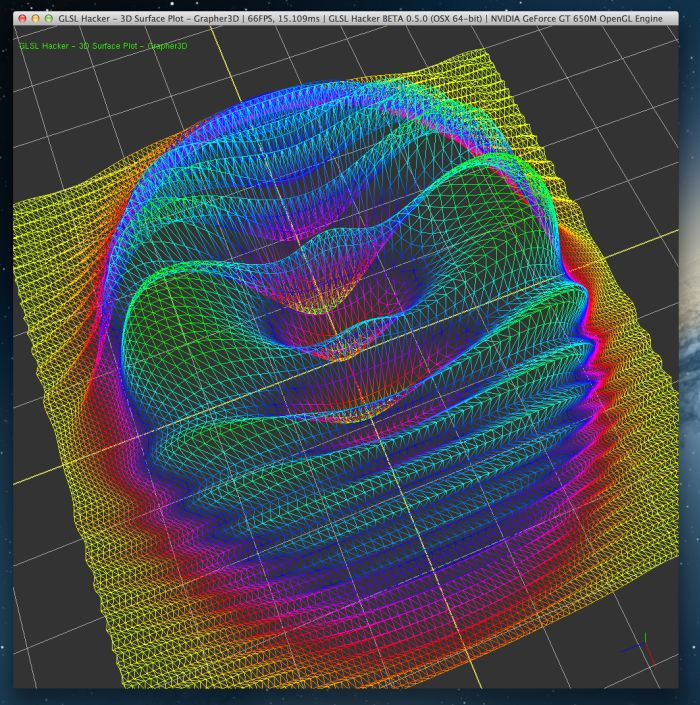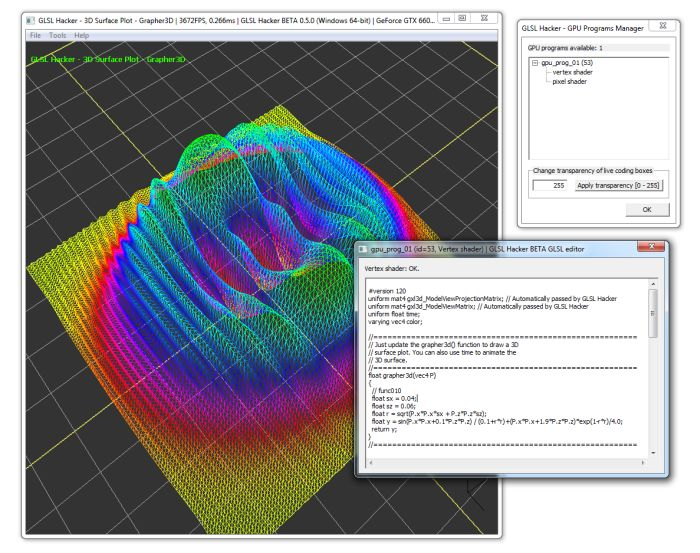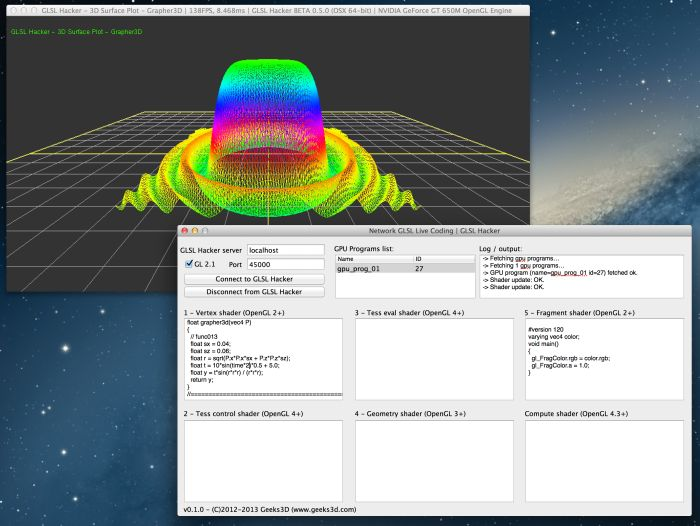# 3D Surfaces Plots (GLSL)Article Index:

## Overview

Here are some equations that allows you to compute surfaces in the 3D space following the general relation: z = f(x, y) or in the case of an OpenGL 3D space (GLSL Hacker for example), y = f(x, z). The following demos are all based on a 100×100-vertex mesh grid that is deformed by a GLSL vertex shader according to the 3D surface equation.

The demos have been created with GLSL Hacker (Windows / OS X / Linux) and source codes (Lua + GLSL) are available in the 3D_Surface_Plots/ folder of the Code Sample Pack.

The vertex shader also contains a function to convert a HSL color to a RGB color. I adapted this routine in Javascript in GLSL. The hslToRgb() function allows to create the cool color transition according to the vertex height.

You can also use GLSL Hacker as an interactive 3D surface editor by editing the vertex shader with the live-coding tool:Live coding under WindowsLive coding under Mac OS X

As usual, the different GLSL shaders are not specific to GLSL Hacker and can be used in any OpenGL application.

Here is the vertex shader used by all demos. The grapher3d() function contains the equation of the 3D surface.

```#version 120

// Automatically passed by GLSL Hacker
uniform mat4 gxl3d_ModelViewProjectionMatrix;

uniform float time;
varying vec4 color;

//========================================================
// Just update the grapher3d() function to draw a 3D
// surface plot. You can also use time to animate the
// 3D surface.
//========================================================

float grapher3d(vec4 P)
{
// func001
float s = 0.5;
float y = cos(abs(P.x)*s+abs(P.z)*s)*(abs(P.x)*s+abs(P.z)*s);
return y;
}

//========================================================

float hue2rgb(float p, float q, float t)
{
float tt = t;
if (tt < 0.0) tt += 1.0;
if (tt > 1.0) tt -= 1.0;
if (tt < 1.0/6.0) return p + (q - p) * 6.0 * tt;
if (tt < 1.0/2.0) return q;
if (tt < 2.0/3.0) return p + (q - p) * (2.0/3.0 - tt) * 6.0;
return p;
}

vec3 hslToRgb(float h, float s, float l)
{
float r, g, b;
if(s == 0.0)
{
r = g = b = l; // achromatic
}
else
{
float q;
if (l < 0.5)
q = l * (1.0 + s);
else
q = l + s - l * s;

float p = 2.0 * l - q;
r = hue2rgb(p, q, h + 1.0/3.0);
g = hue2rgb(p, q, h);
b = hue2rgb(p, q, h - 1.0/3.0);
}
return vec3(r, g, b);
}

void main()
{
vec4 P = gl_Vertex;
P.y = grapher3d(P);

float yRange = 6.0;
float yMax = 2.0;
color.rgb = hslToRgb(0.6 * (yMax - P.y) / yRange, 1, 0.5);

gl_Position = gxl3d_ModelViewProjectionMatrix * P;
}
```

Article Index: